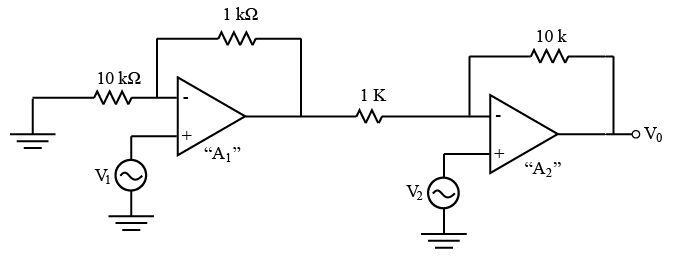# ISRO Scientist or Engineer Electronics Dec 2017

Instructions

For the following questions answer them individually

Question 11

# Relationship between doppler frequency shifts of two radars A and B having 0.1 foot and 0.05 foot wavelengths, approaching the target at 1000 feet per second and 2000 feet per second rate respectively, will beQuestion 12

# A sinusoidal input which can be reproduced in an OP-AMP without any distortion having slew rate of $$10 \pi \frac{V}{\mu s}$$ and 5V peak output amplitude, has the maximum frequency ofQuestion 13

# Which of the following is NOT a characteristic of Schottky Diode?Question 14

# A Sensistor exhibits the following characteristicsQuestion 15

# When an electromagnetic wave is incident on an object having surface roughness comparable to the wavelength, thenQuestion 16

# For the following circuit, determine the output voltage $$‘V_{0}’$$ in terms of input voltages $$V_{1}$$ and $$V_{2}$$ , assuming $$A_{1} and A_{2}$$ are ideal op-ampsQuestion 17

# Dominant mechanism for motion of charge carriers in forward and reverse biased silicon p-n junctions areQuestion 18

# Which of the following is NOT true about opto-couplers?Question 19

# Which of the noise types is dominant in Metal Semiconductor Field Effect Transistor (MESFET) when compared to Bipolar Junction Transistor (BJT)?Question 20

# Which factor determines the range resolution of a radar?OR